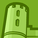Announcements
The way to achieve your own success is the willingness to help somebody else. Go for it!
cancel
Showing results for
Did you mean:Creator

## Bar Chart don't show proper graph

I have created a bar chart with the value list:

ValueList('CY', 'LY')

where CY stands for Current Year and LY stands for Last Year.

for values the below formula is used:

if(ValueList('CY', 'LY')='CY',

SUM(AGGR(if((\$(vSalesLYYTD))>0 and (\$(vSalesYTD))>0, (\$(vSalesYTD)),0),[Unit Key])),

if(ValueList('CY', 'LY')='LY',

SUM(AGGR(if((\$(vSalesLYYTD))>0 and (\$(vSalesYTD))>0, (\$(vSalesLYYTD)),0),[Unit Key]))

)

)

where vSalesLY is:

Sum({\$<[Year] = {"\$(=Year(Today())-1)"} >} [Sales])

and vSalesCY is:

Sum({\$<[Year] = {"\$(=Year(Today()))"} >} [Sales])

The graph is not showing properly. The first bar shows the rights size and figures but for the second bar it just shows zero.

If I switch the values for CY and LY; again the first bar shows the right values whereas the second bar is zero.

10 RepliesPartner - Master III

try This:

Pick(Wildmatch(ValueList('CY', 'LY'),'CY','LY'),

SUM(AGGR(if((\$(vSalesLYYTD))>0 and (\$(vSalesYTD))>0, (\$(vSalesYTD)),0),[Unit Key])),

SUM(AGGR(if((\$(vSalesLYYTD))>0 and (\$(vSalesYTD))>0, (\$(vSalesLYYTD)),0),[Unit Key]))

)Partner - Master III

Or

if(Wildmatch(ValueList('CY', 'LY'),'CY'),

SUM(AGGR(if((\$(vSalesLYYTD))>0 and (\$(vSalesYTD))>0, (\$(vSalesYTD)),0),[Unit Key])),

if(Wildmatch(ValueList('CY', 'LY'),'LY'),

SUM(AGGR(if((\$(vSalesLYYTD))>0 and (\$(vSalesYTD))>0, (\$(vSalesLYYTD)),0),[Unit Key]))

)

)Creator
Author

it brings the same result as beforeCreator
Author

it brings the same result as before. Even if I put the same :

SUM(AGGR(if((\$(vSalesLYYTD))>0 and (\$(vSalesYTD))>0, (\$(vSalesYTD)),0),[Unit Key]))

for both CY and LY, the second bar is zero.Partner - Master III

What does these expression do?

why you are not using

vSalesLY:

Sum({\$<[Year] = {"\$(=Year(Today())-1)"} >} [Sales])

and vSalesCY:

Sum({\$<[Year] = {"\$(=Year(Today()))"} >} [Sales])Creator
Author

If I directly use these statements then the graph shows the right values.

The below statement actually covers a business requirement where we need to show CY Total and LY Total only for those stores (Unit Key) where there is sales in the current year as well as last year. It skip those stores where either last year or the current year sales dont exists.

SUM(AGGR(if((\$(vSalesLYYTD))>0 and (\$(vSalesYTD))>0, (\$(vSalesYTD)),0),[Unit Key]))Partner - Master III

Does this expression work in KPI object?Creator
Author

Yes this works in KPI object. both of them.Partner - Master III

what are the other dimensions you have in chart?Tags
Community Browser